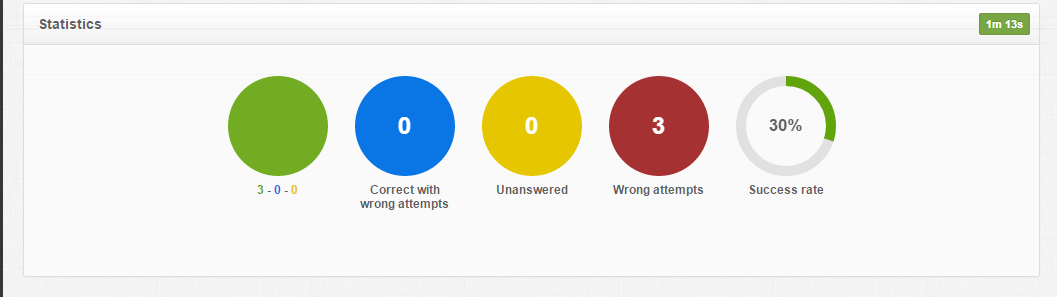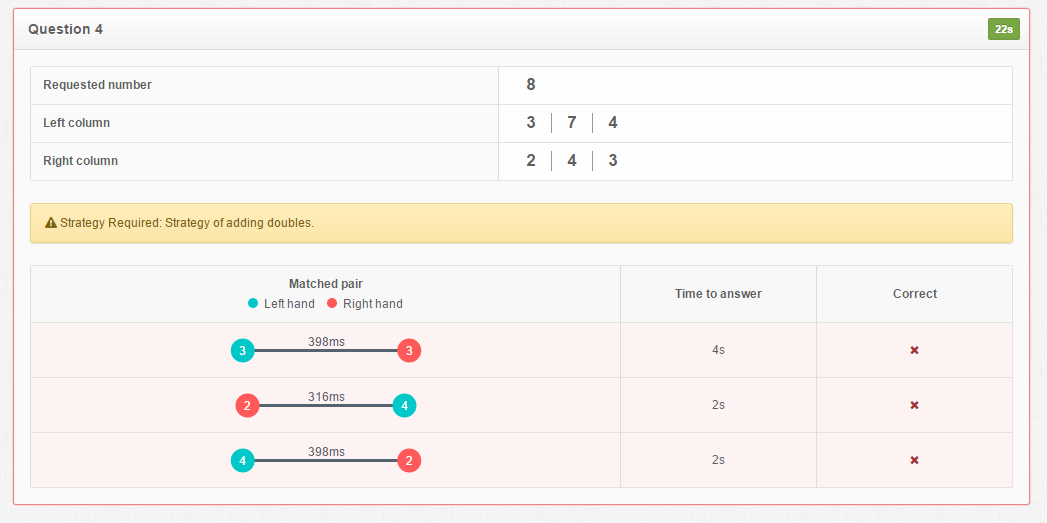### Yummy Pairs

In the “Yummy Pairs” game the student can “decompose” or “deconstruct” numbers that appear as positive integers or quantities using the ten-frame representation. This means they will explore the pairs of number combinations that make up the number. They will also improve their bilateral coordination skills by trying to make symmetrical hand movements.

The avatar represents the student within an imaginary world of candies. The student attempts to match pairs of either numbers or quantities that compose a target number by stretching both hands to reach the two parts that make the whole. The teacher/therapist can select the range of the target number that the child will be asked to make (up to 100) and the difficulty level that involves the available pairs of numbers from which the student will choose. This game offers unique learning and motoric experiences to the children about how numbers can be broken apart and put back together.

Are you ready to choose the right yummy pairs from candy trees?

## Supported goals in Common CoreCaliforniaFloridaNevadaNew YorkNorth CarolinaPennsylvaniaSouth CarolinaTenesseeTexasVirginia

CCSS.MATH.CONTENT.K.OA.A.4
Math
For any number from 1 to 9, find the number that makes 10 when added to the given number, e.g., by using objects or drawings, and record the answer with a drawing or equation.
CCSS.MATH.CONTENT.1.NBT.B.2
Math
Understand that the two digits of a two-digit number represent amounts of tens and ones.
CCSS.MATH.CONTENT.K.NBT.A.1
Math
Compose and decompose numbers from 11 to 19 into ten ones and some further ones, e.g., by using objects or drawings, and record each composition or decomposition by a drawing or equation (such as 18 = 10 + 8); understand that these numbers are composed of ten ones and one, two, three, four, five, six, seven, eight, or nine ones.
CA.MATH.K.OA.4
Math
Understand addition as putting together and adding to, and understand subtraction as taking apart and taking from. For any number from 1 to 9, find the number that makes 10 when added to the given number, e.g., by using objects or drawings, and record the answer with a drawing or equation.
MAFS.K.OA.1.AP.4b
Math
For any number from 1-9, find the number that makes 10 when added to the given number by using objects or drawings
NVAC.MATH.K.OA.A.4
Math
Understand addition as putting together and adding to, and understand subtraction as taking apart and taking from. For any number from 1 to 9, find the number that makes 10 when added to the given number, e.g., by using objects or drawings, and record the answer with a drawing or equation.
NY.MATH.K.OA.4
Math
For any number from 1 to 9, find the number that makes 10 when added to the given number, e.g., by using objects or drawings, and record the answer with a drawing or equation.
NC.MATH.K.OA.4
Math
For any number from 0 to 10, find the number that makes 10 when added to the given number using objects or drawings, and record the answer with a drawing or expression.
PA.CC.MATH.1.NBT.B.2
Math
Understand that the two digits of a two-digit number represent amounts of tens and ones.
PA.CC.MATH.K.OA.A.4
Math
For any number from 1 to 9, find the number that makes 10 when added to the given number, e.g., by using objects or drawings, and record the answer with a drawing or equation.
PA.CC.MATH.K.NBT.A.1
Math
Compose and decompose numbers from 11 to 19 into ten ones and some further ones, e.g., by using objects or drawings, and record each composition or decomposition by a drawing or equation (such as 18 = 10 + 8); understand that these numbers are composed of ten ones and one, two, three, four, five, six, seven, eight, or nine ones.
SC.MATH.K.ATO.4
Math
Create a sum of 10 using objects and drawings when given one of two addends 1 – 9.
No supported goals.
TEKS.MATH.K.5.A
Math
Recite numbers up to at least 100 by ones and tens beginning with any given number.
TEKS.MATH.1.2.B
Math
Use concrete and pictorial models to compose and decompose numbers up to 120 in more than one way as so many hundreds, so many tens, and so many ones;
VASOL.MATH.K.CE.6
Math
The student will model adding and subtracting whole numbers, using up to 10 concrete objects.

## Settings

### I CAN statement

Music

Disable background music.

• On
• Off
Sound effects

Disable/enable sound effects

• On
• Off

I CAN understand that there are sounds when I pick a correct or a wrong combination of numbers/quantities.

Select hand
• Left
• Right

I CAN select the “Play Bottom” with my left hand.

Time to select item

Time to remain stable to select the correct answer.

Reduce the time if the child shows arm stability & good eye-hand coordination.

• 0.5 sec
• 1 sec
• 1.5 sec
• 2 secs
• 2.5 secs
• 3 secs

I CAN select an answer, holding my right hand still for 3 seconds.

Timer

Time to match the right pairs that compose the requested number.

Disable this if the student feels pressure.

• On
• Off

I CAN match the correct pairs of candies under the pressure of time.

Timer value
• 10 secs
• 20 secs
• 30 secs
• 40 secs
• 50 secs
• 60 secs
Game lives

Disable this to continue playing without losing lives when making mistakes.

• On
• Off

I CAN learn that if I get an answer wrong, my game lives will be decreased.

Number of questions

Set how many questions you want the students to practice.

• 5 questions
• 10 questions
• 15 questions

I CAN stay focused for 10 questions.

Number mode

Teachers can determine if the matching pairs will be numeric or quantitative.

• Quantity
• Numeral
• Tens - ones
• Tens - ones (shuffled)
• Tens - ones (reversed)

I CAN compose numbers from two pairs of quantities.

Show numbers

This option shows the quantities in numbers facilitating the composing of quantities.

• On
• Off

I CAN compose quantities that are accompanied by numbers.

Game difficulty

This option makes it harder to compose numbers using various strategies in an automatic way (such as ten-base structure and halves).

• Easy
• Hard

I CAN compose numbers using the ten-base structure.

Content language
• English
• Greek
• Spanish
Numeric range

Adjust the range to match the student’s abilities.

• 2 - 10
• 11 - 20
• 20 - 100
• 11 - 99

I CAN compose two one-digit numbers.

avatar
• 0
• 1
• 2
• 3
• 4
• 5
• 6
• 7

I CAN control the movements of the child in the game with my own body movements.

## ReportsPie chart data

This shows statistics of how many times the student gave correct & wrong or no answers how many times the student tried to find the correct answers

The Time is shown on the top right corner. The teacher can use the time to tell whether the task is challenging enough for the students.Graph data

This table shows the time that was passed from selecting a number/quantity from the right and left side. Particularly, it shows the time between left hand (blue circle) and right hand (red circle) for all the attempts. It is clear that this student took the same time trying to select a number using his left hand compared to his right hand. The required strategy of adding doubles was not used and child gave three wrong answers.